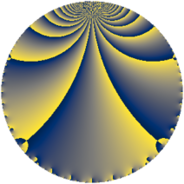# Properties

 Label 1344.4.qLevel $1344$ Weight $4$ Character orbit 1344.q Rep. character $\chi_{1344}(193,\cdot)$ Character field $\Q(\zeta_{3})$ Dimension $192$ Sturm bound $1024$

# Related objects

## Defining parameters

 Level: $$N$$ $$=$$ $$1344 = 2^{6} \cdot 3 \cdot 7$$ Weight: $$k$$ $$=$$ $$4$$ Character orbit: $$[\chi]$$ $$=$$ 1344.q (of order $$3$$ and degree $$2$$) Character conductor: $$\operatorname{cond}(\chi)$$ $$=$$ $$7$$ Character field: $$\Q(\zeta_{3})$$ Sturm bound: $$1024$$

## Dimensions

The following table gives the dimensions of various subspaces of $$M_{4}(1344, [\chi])$$.

Total New Old
Modular forms 1584 192 1392
Cusp forms 1488 192 1296
Eisenstein series 96 0 96

## Trace form

 $$192q - 864q^{9} + O(q^{10})$$ $$192q - 864q^{9} + 144q^{13} + 240q^{21} - 2400q^{25} + 800q^{29} + 488q^{37} + 752q^{53} - 2160q^{61} + 3504q^{77} - 7776q^{81} + 4704q^{85} + 1848q^{93} - 2976q^{97} + O(q^{100})$$

## Decomposition of $$S_{4}^{\mathrm{new}}(1344, [\chi])$$ into newform subspaces

The newforms in this space have not yet been added to the LMFDB.

## Decomposition of $$S_{4}^{\mathrm{old}}(1344, [\chi])$$ into lower level spaces

$$S_{4}^{\mathrm{old}}(1344, [\chi]) \cong$$ $$S_{4}^{\mathrm{new}}(7, [\chi])$$$$^{\oplus 14}$$$$\oplus$$$$S_{4}^{\mathrm{new}}(14, [\chi])$$$$^{\oplus 12}$$$$\oplus$$$$S_{4}^{\mathrm{new}}(21, [\chi])$$$$^{\oplus 7}$$$$\oplus$$$$S_{4}^{\mathrm{new}}(28, [\chi])$$$$^{\oplus 10}$$$$\oplus$$$$S_{4}^{\mathrm{new}}(42, [\chi])$$$$^{\oplus 6}$$$$\oplus$$$$S_{4}^{\mathrm{new}}(56, [\chi])$$$$^{\oplus 8}$$$$\oplus$$$$S_{4}^{\mathrm{new}}(84, [\chi])$$$$^{\oplus 5}$$$$\oplus$$$$S_{4}^{\mathrm{new}}(112, [\chi])$$$$^{\oplus 6}$$$$\oplus$$$$S_{4}^{\mathrm{new}}(168, [\chi])$$$$^{\oplus 4}$$$$\oplus$$$$S_{4}^{\mathrm{new}}(224, [\chi])$$$$^{\oplus 4}$$$$\oplus$$$$S_{4}^{\mathrm{new}}(336, [\chi])$$$$^{\oplus 3}$$$$\oplus$$$$S_{4}^{\mathrm{new}}(448, [\chi])$$$$^{\oplus 2}$$$$\oplus$$$$S_{4}^{\mathrm{new}}(672, [\chi])$$$$^{\oplus 2}$$